# Learn C Programming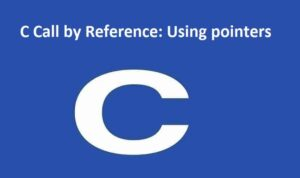#### C Call by Reference: Using pointers

C Call by Reference: Using pointers In this tutorial, you'll learn to pass addresses as arguments to the function with ...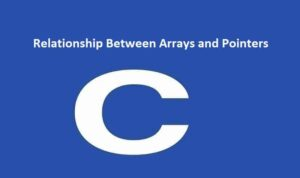#### Relationship Between Arrays and Pointers

In this tutorial, you'll find out about the relationship between arrays and pointers in C programming. You will also learn ...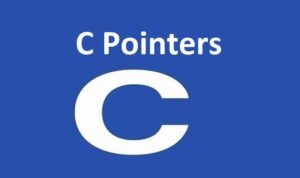#### C Pointers

C Pointers : In this tutorial, you'll find out about pointers; what pointers are, how would you use them, and ...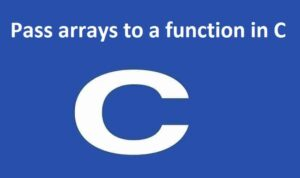#### Pass arrays to a function in C

In this tutorial, you'll learn to pass arrays (both one-dimensional and multidimensional arrays) to a function in C programming with ...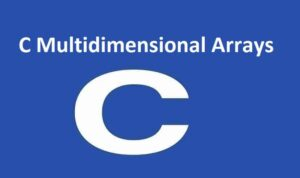#### C Multidimensional Arrays

In this tutorial, you will learn to function with multidimensional arrays (two-dimensional and three-dimensional array) with the help of an ...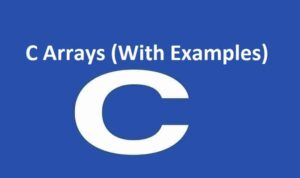#### C Arrays

C Arrays In this tutorial, you will learn to function with arrays. You will learn to declare, introduce, and access ...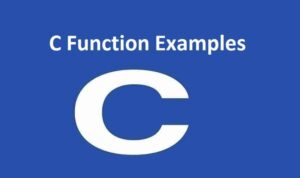#### C Function Examples

C Function Examples In this article, you will discover a rundown of C programs to sharpen your knowledge of functions ...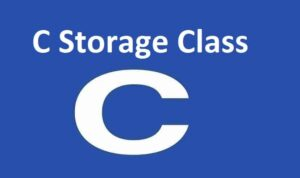#### C Storage Class

C Storage Class In this tutorial, you will find out about the scope and lifetime of local and global variables ...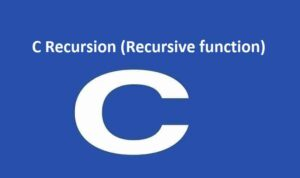#### C Recursion

C Recursion C Recursion: In this tutorial, you will learn to write recursive functions in C programming with the help ...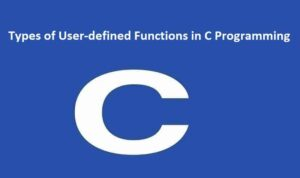#### Types of User-defined Functions in C Programming

Types of User-defined Functions in C Programming In this tutorial, you will find out about various approaches you can take ...